Math resources Geometry Angles

Students will first learn about adjacent angles as part of geometry and measurement and data in 4 th grade.

Adjacent angles are two angles that are side by side and share a common vertex and a common side. They are often formed by intersecting lines or line segments.

Since an angle is formed when two rays meet at a common endpoint, adjacent angles are simply two angles that are directly next to each other. Adjacent angles can be complementary angles or supplementary angles.

For example, in this diagram, angle XWY is adjacent to angle YWZ . They share a common vertex ( W ) and a common side (ray WY ).

### Types of angles

A linear pair is a pair of adjacent angles that combine to form a straight angle. The angles in a linear pair are supplementary angles, meaning their measures add up to 180^{\circ}.

Adjacent angles can also be complementary angles. Complementary adjacent angles are angles that add up to 90^{\circ} .

Adjacent angles can also be found within the interior angles of polygons.

For example, one pair of adjacent angles in the pentagon is angle RQU and angle QUT .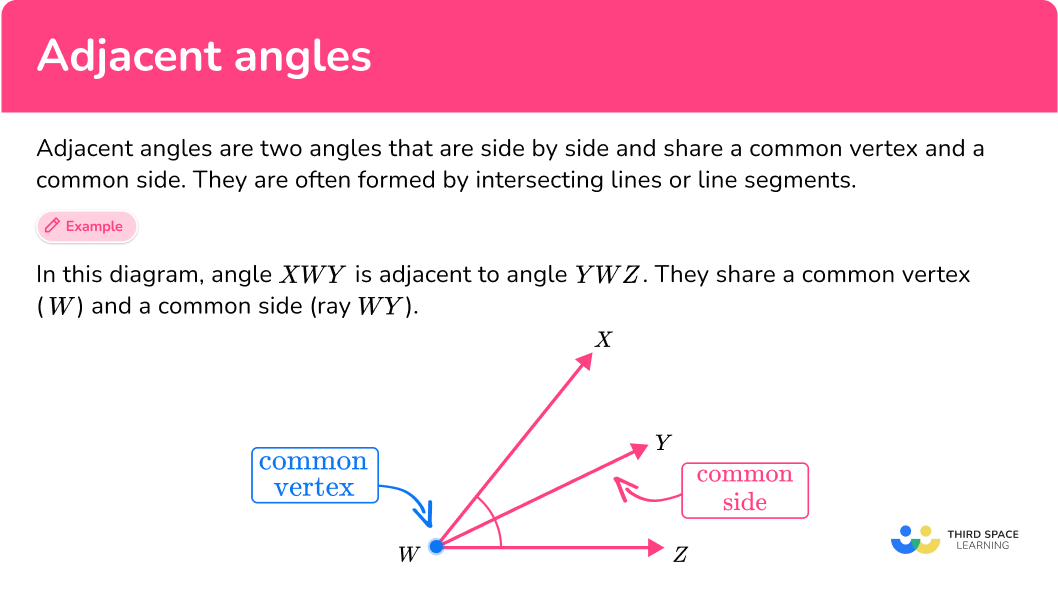## Common Core State Standards

How does this relate to 4th grade math?

• Grade 4 – Geometry (4.G.A.1)
Draw points, lines, line segments, rays, angles (right, acute, obtuse), and perpendicular and parallel lines. Identify these in two-dimensional figures.

• Grade 4 – Geometry (4.MD.C.7)
Recognize angle measure as additive. When an angle is decomposed into non-overlapping parts, the angle measure of the whole is the sum of the angle measures of the parts. Solve addition and subtraction problems to find unknown angles on a diagram in real world and mathematical problems, for example, by using an equation with a symbol for the unknown angle measure.

## How to identify adjacent angles

In order to identify adjacent angles:

1. Recall the definition of adjacent angles.
2. Determine which angles are adjacent and name them.

### Example 1: identify adjacent angles

Name the pair of adjacent angles.

1. Recall the definition of adjacent angles.

Adjacent angles are two angles that are side by side and share a common vertex and a common side.

2Determine which angles are adjacent and name them.

Angle SHG and angle GHV are adjacent angles.

### Example 2: identify adjacent angles

Name the acute angle that is adjacent to angle QPE .

Adjacent angles are two angles that are side by side and share a common vertex and a common side.

Angle EPH is adjacent to angle QPE .

### Example 3: identify multiple pairs of adjacent angles

Name 2 pairs of adjacent angles.

Adjacent angles are two angles that are side by side and share a common vertex and a common side.

Any angles that share a common vertex and a common side are adjacent angles. For example, angle DZC and angle CZO are one pair of adjacent angles. Another pair of adjacent angles is angle CZO and angle OZF .

### Example 4: identify adjacent angles in real world objects

Find two pairs of adjacent angles in the pizza.

Adjacent angles are two angles that are side by side and share a common vertex and a common side.

Any angles that share a common vertex and a common side are adjacent angles. For example, one pair of adjacent angles in the pizza is angle CZD and angle DZE . Another pair of adjacent angles is angle GZH and angle HZA .

## How to find a missing angle measure within adjacent angles

In order to find a missing angle measure within adjacent angles:

1. Recall the definition of adjacent angles.
2. Determine which angles are adjacent and name them.
3. Subtract the known angle measure from the total angle measure of the adjacent angles.

### Example 5: find the missing angle measure.

The total angle measure of the adjacent angles is 130^{\circ} . What is the measure of angle XBK?

Adjacent angles are two angles that are side by side and share a common vertex and a common side.

The adjacent angles are angle RBX and angle BXK .

Total angle measure = 130^{\circ} .

Known angle measure (angle RBX ) = 50^{\circ}

130^{\circ}-50^{\circ} = 80^{\circ}

So the missing angle measure (angle BXK ) is 80^{\circ} .

### Example 6: find the missing angle measure in a linear pair of angles.

A linear pair of angles is a pair of adjacent angles that add up to 180^{\circ} . They are also supplementary angles.

What is the measure of angle JUP?

Adjacent angles are two angles that are side by side and share a common vertex and a common side.

The adjacent angles are angle JUP and angle PUL .

Total angle measure = 180^{\circ} .

Known angle measure (angle PUL ) = 45^{\circ}

180^{\circ}-45^{\circ} = 135^{\circ}

So the missing angle measure (angle JUP ) is 135^{\circ} .

### Teaching tips for adjacent angles

• Providing real-life examples of adjacent angles (either hands-on or on worksheets) to make the concept more relatable and tangible for students. For instance, you can use classroom objects, such as books or desks.

• Incorporate interactive games or online resources that focus on adjacent angles. There are numerous educational websites and apps that provide interactive activities where students can practice identifying, measuring, and classifying adjacent angles.

### Easy mistakes to make

• Overlapping angles
Students may believe that if two angles overlap partially or fully, they are considered adjacent angles. However, adjacent angles should not overlap; they share a common vertex and a common side but do not intersect.
For example, angle MLO is not adjacent to angle MLN since they overlap.

• Non-adjacent angles on the same line
Students may confuse non-adjacent angles on the same line as adjacent angles. It’s important to clarify that adjacent angles must have a common vertex and side, meaning they are next to each other.
For example, angle ABE and angle DBC are located on the same straight line, but they are not adjacent angles since they are not next to each other.

Related angles lessons

1) Which pair of angles are adjacent?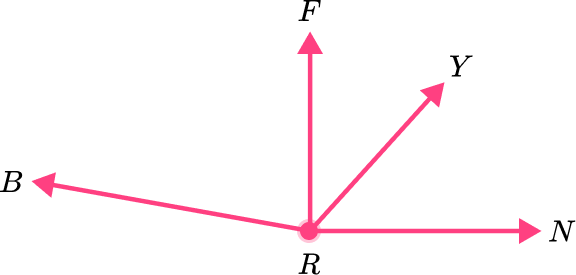angle BRF and angle RYNangle FRY and angle YRNangle BRF and angle BRYangle YRN and angle FRNAngle FRY and angle YRN are adjacent angles since they are next to each other without overlapping.

2) Which angle is adjacent to angle COB ?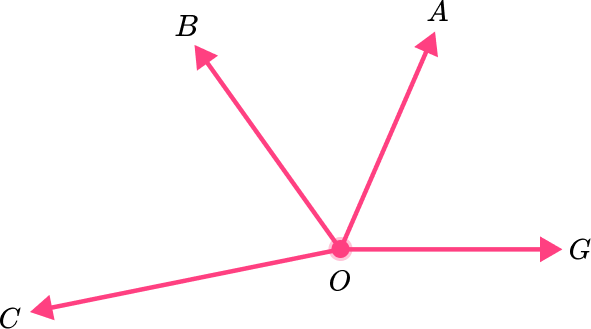angle AOGangle COAangle BOAangle BAGAngle BOA is right next to angle COB and they do not overlap, so they are adjacent.

3) Name two pairs of adjacent angles on the clock.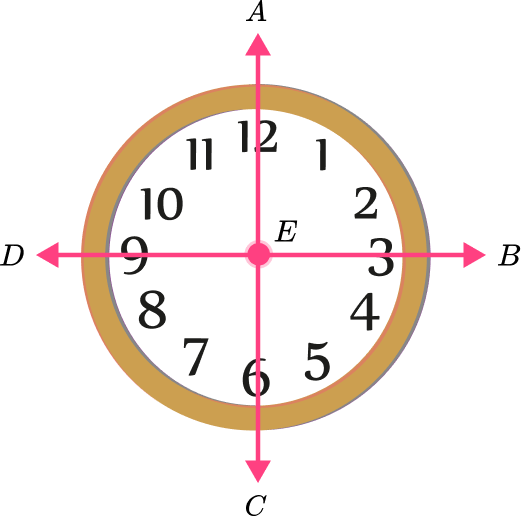Angles AEB and AEC ; angles AEB and BECAngles CED and CEA ; angles DEA and AEBAngles DEB and BED ; angles AEC and BECAngles BEC  and CED ; angles AEB and BECOne pair of adjacent angles is angle BEC and angle CED . Another pair is angle AEB and angle BEC . These are the only options in the answer choices where each pair of angles does not overlap.

4) The adjacent angles shown below are complementary angles, so together they equal 90^{\circ} . What is the missing angle measure?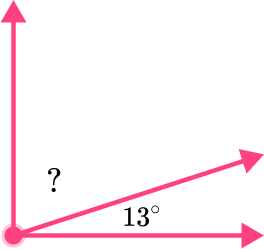90^{\circ}77^{\circ}167^{\circ}13^{\circ}Since you know the total measure of the adjacent angles is 90^{\circ} , you can subtract 13^{\circ} from 90^{\circ} .

90^{\circ}-13^{\circ}=77^{\circ}

5) What is the missing angle measure in the linear pair of angles?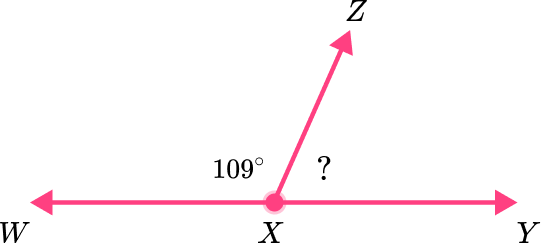71^{\circ}81^{\circ}180^{\circ}19^{\circ}A linear pair is a pair of adjacent angles that combine to form a straight angle. The angles in a linear pair are supplementary angles, meaning their measures add up to 180^{\circ} . To calculate the missing angle, we subtract the known angle from the total angle measure of 180^{\circ} .

180^{\circ}-109^{\circ}=71^{\circ}

6) Name a pair of adjacent angles in the polygon.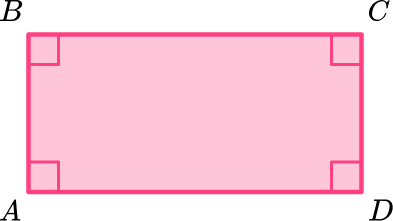Angle A and angle CAngle C and angle DAngle B and angle DThere are no adjacent angles in this polygon.Angle C and angle D are adjacent angles because they are interior angles in a polygon (specifically a rectangle) that are right next to each other. They are also both right angles.

Adjacent angles are two angles that are side by side and share a common vertex and a common side.

What are the properties of adjacent angles?

The properties of adjacent angles are:
◌ they share a common vertex
◌ they share a common side
◌ they do not overlap
◌ they can be part of a linear pair or have other angle relationships like being complementary or supplementary

What is a linear pair of angles?

A linear pair is a pair of adjacent angles that combine to form a straight angle. The angles in a linear pair are supplementary angles, meaning their measures add up to 180^{\circ}.

When are adjacent angles complementary angles?

Adjacent angles are complementary when the sum of their measures is equal to 90^{\circ}.

When are adjacent angles supplementary angles?

Adjacent angles are supplementary when the sum of their measures is equal to 180^{\circ}.

Can vertical angles be adjacent angles?

No, vertical angles cannot be adjacent angles. Vertical angles are formed when two lines intersect, and they are opposite to each other. Adjacent angles are next to each other.

## Still stuck?

At Third Space Learning, we specialize in helping teachers and school leaders to provide personalized math support for more of their students through high-quality, online one-on-one math tutoring delivered by subject experts.

Each week, our tutors support thousands of students who are at risk of not meeting their grade-level expectations, and help accelerate their progress and boost their confidence.